# Texas Go Math Grade 4 Lesson 2.7 Answer Key Order Decimals

Refer to our Texas Go Math Grade 4 Answer Key Pdf to score good marks in the exams. Test yourself by practicing the problems from Texas Go Math Grade 4 Lesson 2.7 Answer Key Order Decimals.

## Texas Go Math Grade 4 Lesson 2.7 Answer Key Order Decimals

Essential Question

How can you order decimals?
The steps for ordering decimals are:
a. Set up a table with the decimal point in the same place for each number.
b. Put in each number.
c. Fill in the empty squares with zeros.
d. Compare using the first column on the left.
e. If the digits are equal move to the next column to the right until one number wins.

Investigate

Materials

• string
• clothespins
• marker
• index cards

A. Use your marker to mark the location of benchmark decimals 1.0, 1.5, and 2.0 on your string.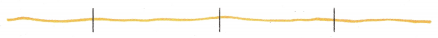B. Use clothespins and index cards to label the points you marked.C. Now locate the points 1 .2, 1.9, and 1.6 on your string by using clothespins and labeled index cards.D. Draw a picture of the number line you modeled.E. Compare your model with another student’s model. Do either of you want to change any of the positions of your clothespins? Explain.Now,
When we compare the both models,
We can observe that both the models are the same
Hence, from the above,
We can conclude that
Neither you nor your friend has to change any of the positions of your clothespins

Make Connections

You can also use place value to order decimals.

Order $1.52,$0.87, and $1.56 from least to greatest.Hence, from the above, We can conclude that The order from least to greatest is: 0.87, 1.52, and 1.56 Math Talk MathematicaI Processes How is ordering decimals similar to comparing decimals? Answer: Decimal numbers are compared in the same way as other numbers: by comparing the different place values from left to right. We use the symbols <, > and = to compare decimals as shown below. When comparing two decimals, it is helpful to write one below the other. Share and Grow Use the number line to order the decimals from least to greatest.Question 1. 1.9, 1.09, 1.5, 1.55 Answer: The given decimal numbers are: 1.9, 1.09, 1.5, and 1.55 Now, The given number line is:Now, The representation of the decimal numbers in the number line is:Now, We know that, The numbers that are close to zero (or) to the starting point will be the least numbers The numbers that are farther to zero (or) to the starting point will be the least numbers Now, From the above number line, We can observe that 1.09 is the closest to the starting line of the number line So, 1.09 is the least number After 1.09. The least number will be: 1.50 After 1.50, The lowest number will be: 1.55 Hence. from the above, We can conclude that The order of the numbers from the least to the greatest is: 1.09, 1.50, 1.55, and 1.90 Texas Go Math Grade 4 Lesson 2.7 Answer Key Question 2. 1.65, 1.56, 1.6, 2.0 Answer: The given decimal numbers are: 1.65, 1.56, 1.6, and 2.0 Now, The given number line is:Now, The representation of the decimal numbers in the number line is:Now, We know that, The numbers that are close to zero (or) to the starting point will be the least numbers The numbers that are farther to zero (or) to the starting point will be the least numbers Now, From the above number line, We can observe that 1.56 is the closest to the starting line of the number line So, 1.56 is the least number After 1.56. The least number will be: 1.60 After 1.60, The least number will be: 1.65 Hence. from the above, We can conclude that The order of the numbers from the least to the greatest is: 1.56, 1.60, 1.65, and 2.0 Order the decimals from greatest to least. You can use place value or a number line on your Math Board. Question 3.$1.41, $0.14,$1.14, $1.40 Answer: The given decimal numbers are:$1.41, $0.14,$1.14, and $1.40 Now, We know that, The numbers that are close to zero (or) to the starting point will be the least numbers The numbers that are farther to zero (or) to the starting point will be the least numbers Now, From the given decimal numbers, We can observe that 0.14 is the closest to 0 So, 0.14 is the least number After 0.14. The least number will be: 1.14 After 1.14, The least number will be: 1.40 Hence. from the above, We can conclude that The order of the numbers from the greatest to the least is:$1.41, $1.40,$1.14, and $0.14 Question 4. 7.03, 7.3, 6.98, 6.89 Answer: The given decimal numbers are: 7.03, 7.3, 6.98, and 6.89 Now, We know that, The numbers that are close to zero (or) to the starting point will be the least numbers The numbers that are farther to zero (or) to the starting point will be the least numbers Now, From the given decimal numbers, We can observe that 6.89 is the closest to 0 So, 6.89 is the least number After 6.89. The least number will be: 6.98 After 6.98, The least number will be: 7.03 Hence. from the above, We can conclude that The order of the numbers from the greatest to the least is: 7.3, 7.03, 6.98, and 6.89 Order the decimals from least to greatest. You can use place value or a number line on your MathBoard. Question 5.$1.35,$3.15,$1.53,$3.51 Answer: The given decimal numbers are:$1.35, $3.15,$1.53, and $3.51 Now, We know that, The numbers that are close to zero (or) to the starting point will be the least numbers The numbers that are farther to zero (or) to the starting point will be the least numbers Now, From the given decimal numbers, We can observe that 1.35 is the closest to 0 So, 1.35 is the least number After 1.35. The least number will be: 1.53 After 1.53, The least number will be: 3.15 Hence. from the above, We can conclude that The order of the numbers from the least to the greatest is:$1.35, $1.53,$3.15, and $3.51 Go Math Grade 4 Lesson 2.7 Answer Key Question 6. 6.25,7.2,6.93,7.11 Answer: The given decimal numbers are: 6.25, 7.2, 6.93, and 7.11 Now, We know that, The numbers that are close to zero (or) to the starting point will be the least numbers The numbers that are farther to zero (or) to the starting point will be the least numbers Now, From the given decimal numbers, We can observe that 6.25 is the closest to 0 So, 6.25 is the least number After 6.25. The least number will be: 6.93 After 6.93, The least number will be: 7.11 Hence. from the above, We can conclude that The order of the numbers from the least to the greatest is: 6.25, 6.93, 7.11, and 7.2 Unlock the Problem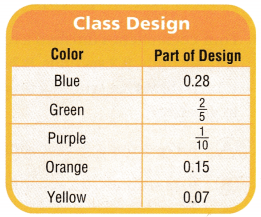Question 7. H.O.T. Multi-Step Martin’s class drew a design using 10 by 10 grid paper. The table shows how much of each color was used in the design. Which color was the third-greatest part of the design? a. What do you need to know? Answer: It is given that Martin’s class drew a design using 10 by 10 grid paper. The table shows how much of each color was used in the design Now, The given table is:Hence, from the above, We can conclude that You need to know the order of the part of the color from the greatest to the least b. Describe a strategy you could use to order the values in the table. Answer: The given table is:So, The strategy you could use to order the values in the table is: Convert the fractions into decimals and order the numbers from the greatest to the least c. How might you use models to help you? Answer: If we use a Number line, Place the numbers on the number line If we use a Rectangular model, Shade the numerator part of the part of the color and order the shaded parts from the greatest to the least d. Show your work. Answer: It is given that Martin’s class drew a design using 10 by 10 grid paper. The table shows how much of each color was used in the design Now, The given table is:Now, From the above table, We can observe that The representation of the decimal numbers present in the given table is: 0.28, 0.4, 0.1, 0.15, and 0.07 Now, From the above decimal numbers, We can observe that 0.1 is the closest to 0 So, 0.1 is the least number 0.4 is the farthest to 0 So, 0.4 is the greatest number Hence, from the above, We can conclude that The order of the part of the color from the greatest to the least is: 0.4, 0.28, 0.15, 0.1, and 0.07 e. Complete the sentences. The greatest part of the design was the color ______________ . The least part of the design was the color ______________ . The third-greatest part of the design was the color ______________ . Answer: From part (d), The order of the part of the color from the greatest to the least is: 0.4, 0.28, 0.15, 0.1, and 0.07 Hence, from the above, We can conclude that The greatest part of the design was the color “Green” . The least part of the design was the color “Yellow” . The third-greatest part of the design was the color ‘Orange” . Go Math Grade 4 Lesson 2.7 Order Decimals Question 8. H.O.T. Use Symbols Howard studied math for 0.75 hours. Carol studied math for 0.80 hours. Who studied math for a greater amount of time? Explain. Answer: It is given that Howard studied math for 0.75 hours. Carol studied math for 0.80 hours Now, The given decimal numbers are: 0.75 and 0.80 Now, The representation of 0.75 and 0.80 in the place-value chart is:Now, From the above place-value chart, We can observe that 7 tenths < 8 tenths So, 0.75 < 0.80 Hence, from the above, We can conclude that Carol studied math for the greater amount of time Question 9. Mark has$3.23, Jen has $3.23, and Sally has$3.32. Who has the most money?
It is given that
Mark has $3.23, Jen has$3.23, and Sally has $3.32 Now, The given decimal numbersa re: 3.23 and 3.32 Now, The representation of 3.23 and 3.32 in the place-value chart is: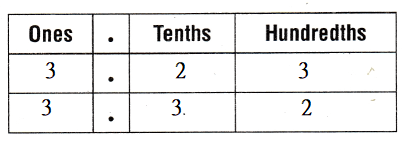Now, From the above place-value chart, We can observe that 2 tenths < 3 tenths So, 3.23 < 3.32 Hence, from the above, We can conclude that Sally has the most money Daily Assessment Task Fill in the bubble completely to show your answer. Question 10. Four cockroaches finished a race. Their winning times are 9.42 seconds, 8.3 seconds, 9.2 seconds, and 8.17 seconds. Which shows the winning times written in order from least to greatest? (A) 8.17 seconds, 8.3 seconds, 9.42 seconds, 9.2 seconds (B) 9.42 seconds, 9.2 seconds, 8.3 seconds, 8.17 seconds (C) 8.17 seconds, 8.3 seconds, 9.2 seconds, 9.42 seconds (D) 9.42 seconds, 8.3 seconds, 9.2 seconds, 8.17 seconds Answer: It is given that Four cockroaches finished a race. Their winning times are 9.42 seconds, 8.3 seconds, 9.2 seconds, and 8.17 seconds Now, The given decimal numbers are: 9.42, 8.3, 9.2, and 8.17 Now, From the given numbers, We can observe that 8.17 is the closest to 0 when compared to the other given numbers So, 8.17 is the least number 9.42 is the farthest to 0 when compared to the other given numbers So, 9.42 is the greatest number Hence, from the above, We can conclude that The winning times written in order from the least to the greatest is:Go Math 4th Grade Lesson 2.7 Order Decimals Question 11. Use place value to order 5.87, 6.14, 5.78, and 6.04. Which shows, the decimals written in order from least to greatest? (A) 6.04, 6.14, 5.87, 5.78 (B) 5.78, 5.87, 6.04, 6.14 (C) 5.78, 5.87, 6.14, 6.04 (D) 5.87, 6.04, 6.14, 5.78 Answer: The given decimal numbers are: 5.87, 6.14, 5.78, and 6.04 Now, The representation of the given decimal numbers in the place-value chart is:Hence, from the above, We can conclude that The order of the decimals written in the order from the least to the greatest is: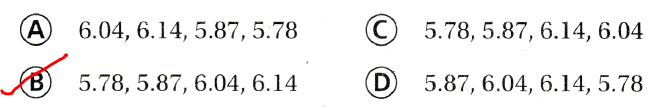Question 12. Representations Use the number line to order the decimals.Which shows the decimals 5.16, 5.28, 5.11, and 5.21 written in order from greatest to least? (A) 5.11,5.16,5.21,5.28 (B) 5.16,5.28,5.11,5.21 (C) 5.21,5.16,5.11,5.28 (D) 5.28,5.21,5.16,5.11 Answer: The given decimal numbers are: 5.16, 5.28, 5.11, and 5.21 Now, The given number line is:Now, The representation of the given decimal numbers on the given number line is: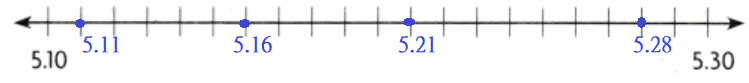Hence, from the above, We can conclude that The order of the given decimal numbers from the greatest to the least is: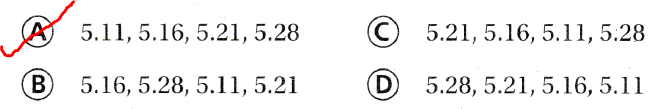TEXAS Test Prep Question 13. Which of the following is less than 14.70? (A) 15.03 (B) 14.09 (C) 14.73 (D) 14.7 Answer: The given decimal number is: 14.70 Now, We know that, 14.70 = 14.7 Now, To represent the decimal number less than 14.70, the tenths place must be less than 7 Hence, from the above, We can conclude that The decimal number that is less than 14.70 is:### Texas Go Math Grade 4 Lesson 2.7 Homework and Practice Answer Key Use the number line to order the decimals from least to greatest.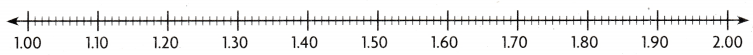Question 1. 1.11, 1.2, 1.01, 1.1 Answer: The given decimal numbers are: 1.11, 1.2, 1.01, and 1.1 Now, The given number line is:Now, The representation of the given decimal numbers on the number line is: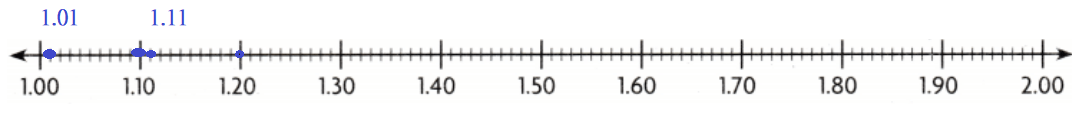Hence, from the above, We can conclude that The order of the given decimal numbers from the least to the greatest is: 1.01, 1.10, 1.11, and 1.20 Go Math Lesson 2.7 4th Grade Practice and Homework Question 2. 1.32,1.23, 1.3, 1.2 Answer: The given decimal numbers are: 1.32, 1.23, 1.3, and 1.2 Now, The given number line is:Now, The representation of the given decimal numbers on the number line is:Hence, from the above, We can conclude that The order of the given decimal numbers from the least to the greatest is: 1.20, 1.23, 1.30, and 1.32 Order the decimals from greatest to least. You can use place value or a number line on your MathBoard. Question 3.$2.15, $1.89,$1.09, $1.90 Answer: The given decimal numbers are:$2.15, $1.89,$1.09, and $1.90 Now, We know that, The numbers that are the closest to 0 are the “Least numbers” The numbers that are the farthest to 0 are the “Greatest numbers” So, From the above, The order of the given numbers from the greatest to the least is:$1.09, $1.89,$1.90. and $2.15 Hence, from the above, We can conclude that The order of the given numbers from the greatest to the least is:$1.09, $1.89,$1.90. and $2.15 Question 4. 0.66, 0.06, 0.60, 0.96 Answer: The given decimal numbers are: 0.66, 0.06, 0.60, and 0.96 Now, We know that, The numbers that are the closest to 0 are the “Least numbers” The numbers that are the farthest to 0 are the “Greatest numbers” So, From the above, The order of the given numbers from the greatest to the least is: 0.96, 0.66, 0.60, and 0.06 Hence, from the above, We can conclude that The order of the given numbers from the greatest to the least is: 0.96, 0.66, 0.60, and 0.06 Problem Solving Question 5. Jamal wrote the following decimals on the board. 4.24, 4.04, 4.18, 4.42 Order these decimals from least to greatest. Answer: It is given that Jamal wrote the following decimals on the board.: 4.24, 4.04, 4.18, 4.42 Now, The given decimal numbers are: 4.24, 4.04, 4.18, and 4.42 Now, We know that, The numbers that are the closest to 0 are the “Least numbers” The numbers that are the farthest to 0 are the “Greatest numbers” So, From the above, The order of the given decimal numbers from the least to the greatest is: 4.04, 4.18, 4.24, and 4.42 Hence, from the above, We can conclude that The order of the given decimal numbers from the least to the greatest is: 4.04, 4.18, 4.24, and 4.42 Lesson 2.7 Answer Key Go Math Grade 4 Question 6. Anna paid$13.32 for a teddy bear. Karl paid $13.02 for a teddy bear. Cindy paid$12.45 for her teddy bear and Mark paid $14.50 for his teddy bear. Order the names from who spent the least to who spent the greatest for their teddy bear. Answer: It is given that Anna paid$13.32 for a teddy bear. Karl paid $13.02 for a teddy bear. Cindy paid$12.45 for her teddy bear and Mark paid $14.50 for his teddy bear Now, The given decimal numbers are:$13.32, $13.02,$12.45, and $14.50 Now, We know that, The numbers that are the closest to 0 are the “Least numbers” The numbers that are the farthest to 0 are the “Greatest numbers” So, From the above, The order of the given decimal numbers from the least to the greatest is:$12.45, $13.02,$13.32, and $14.50 So, The order of the names who spend the least to those who spend the greatest is: Cindy, Karl, Anna, and Mark Hence, from the above, We can conclude that The names of who spent the least to who spent the greatest for their teddy bear is: Cindy, Karl, Anna, and Mark Question 7. During recess, some students ran the 40-yard dash. Tim ran it in 5.64 seconds, Sarah in 5.46 seconds, Hannah in 5.60 seconds, and Jason in 5.49 seconds. Order the times from least to greatest. Answer: It is given that During recess, some students ran the 40-yard dash. Tim ran it in 5.64 seconds, Sarah in 5.46 seconds, Hannah in 5.60 seconds, and Jason in 5.49 seconds Now, The given decimal numbers are: 5.64, 5.46, 5.60, and 5.49 Now, We know that, The numbers that are the closest to 0 are the “Least numbers” The numbers that are the farthest to 0 are the “Greatest numbers” So, From the above, The order of the given decimal numbers from the least to the greatest is: 5.46, 5.49, 5.60, and 5.64 Hence, from the above, We can conclude that The order of the times from the least to the greatest is: 5.46 seconds, 5.49 seconds, 5.60 seconds, and 5.64 seconds Question 8. Karen made four different hats. She used some blue ribbon for each hat. For one hat, she used 0.8 feet of ribbon. For another hat, she used 1.2 feet of ribbon. For the last two hats, she used 1.02 and 1.21 feet of ribbon. Order these amounts from greatest to least. Answer: It is given that Karen made four different hats. She used some blue ribbon for each hat. For one hat, she used 0.8 feet of ribbon. For another hat, she used 1.2 feet of ribbon. For the last two hats, she used 1.02 and 1.21 feet of ribbon Now, The given decimal numbers are: 0.8, 1.2, 1.02, and 1.21 Now, We know that, The numbers that are the closest to 0 are the “Least numbers” The numbers that are the farthest to 0 are the “Greatest numbers” So, From the above, The order of the given decimal numbers from the greatest to the least is: 1.21, 1.2, 1.08, and 0.8 Hence, from the above, We can conclude that The order of the amounts of ribbon from the greatest to the least is: 1.21 feet, 1.2 feet, 1.08 feet, and 0.8 feet Lesson Check Fill in the bubble completely to show your answer. Question 9. Use the number line to order the decimals.Which answer shows 1.31,1.13, 1.3, and 1.1 in order from least to greatest? (A) 1.1,1.3,1.13,1.31 (B) 1.1, 1.13, 1.3, 1.31 (C) 1.13, 1.1, 1.31, 1.3 (D) 1.13,1.31,1.1,1.3 Answer: The given decimal numbers are: 1.31, 1.13, 1.3, and 1.1 Now, The given number line is:Now, The representation of the given decimal numbers on the given number line is: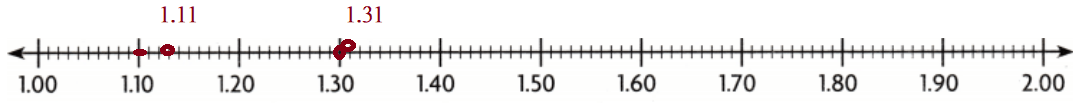Hence, from the above, We can conclude that The order of the given decimal numbers from the least to the greatest is: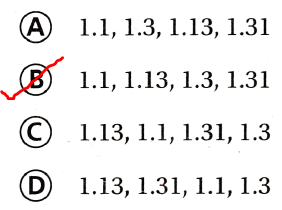Go Math Lesson 2.7 Answer Key 4th Grade Question 10. Order these amounts of money from least to greatest.$4.88, $5.19,$4.83, $5.02 (A)$4.88, $4.83,$5.02, $5.19 (B)$5.19, $5.02,$4.88, $4.83 (C)$4.83, $4.88,$5.02, $5.19 (D)$5.02, $5.19,$4.83, $4.88 Answer: The given amounts of money are:$4.88, $5.19,$4.83, \$5.02
Now,
The given decimal numbers are: 4.88, 5.19, 4.83, and 5.02
Now,
We know that,
The numbers that are the closest to 0 are the “Least numbers”
The numbers that are the farthest to 0 are the “Greatest numbers”
So,
From the above,
The order of the given decimal numbers from the least to the greatest is:
4.83, 4.88, 5.02, and 5.19
Hence, from the above,
We can conclude that
The order of the given amounts of money from the least to the greatest is: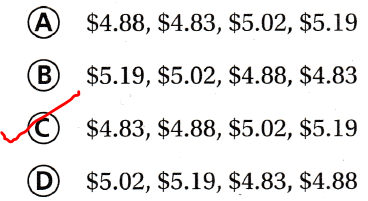Question 11.
Multi-Step Which number is greater than 7.23 and less than 7.55?
(A) 7.59
(B) 7.17
(C) 7.6
(D) 7.3
The given decimal numbers are: 7.23 and 7.55
Now,
To represent the numbers that are greater than 7.23 and less than 7.55,
The numbers should be: 7.24 to 7.54
Hence, from the above,
We can conclude that
The number that is greater than 7.23 and less than 7.55 is: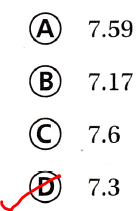Question 12.
Multi-Step Cory, Margo, and Alyssa get the same allowance. Cory saved $$\frac{2}{5}$$ of his allowance. Margo saved 0.35 of hers. Alyssa saved 0.3 of hers. Which statement is true?
(A) Cory saved the most.
(B) Alyssa saved more than Margo.
(C) Cory and Alyssa saved the same amount.
(D) Margo saved more than Cory.
It is given that
Cory, Margo, and Alyssa get the same allowance. Cory saved $$\frac{2}{5}$$ of his allowance. Margo saved 0.35 of hers. Alyssa saved 0.3 of hers
Now,
The representation of $$\frac{2}{5}$$ in the form of a fraction is: 0.4
Now,
The given decimal numbers are: 0.4, 0.35, and 0.3
Now,
We know that,
The numbers that are the closest to 0 are the “Least numbers”
The numbers that are the farthest to 0 are the “Greatest numbers”
So,
From the above,
The order of the given decimal numbers from the least to the greatest is:
0.3, 0.35, and 0.4
Hence, from the above,
We can conclude that
The statement that is true is: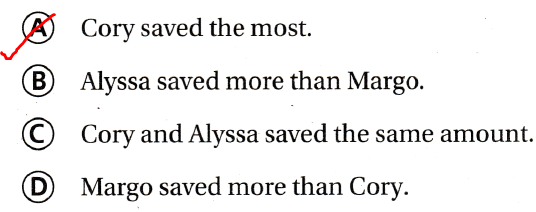Scroll to Top